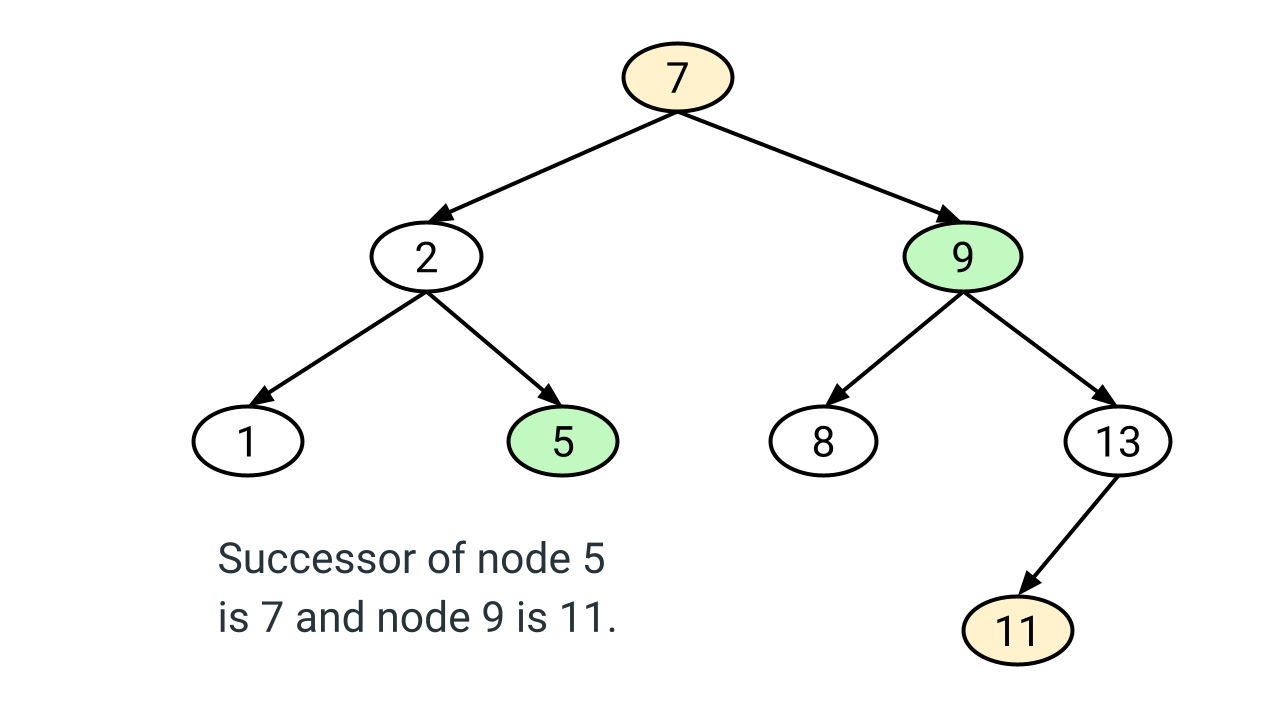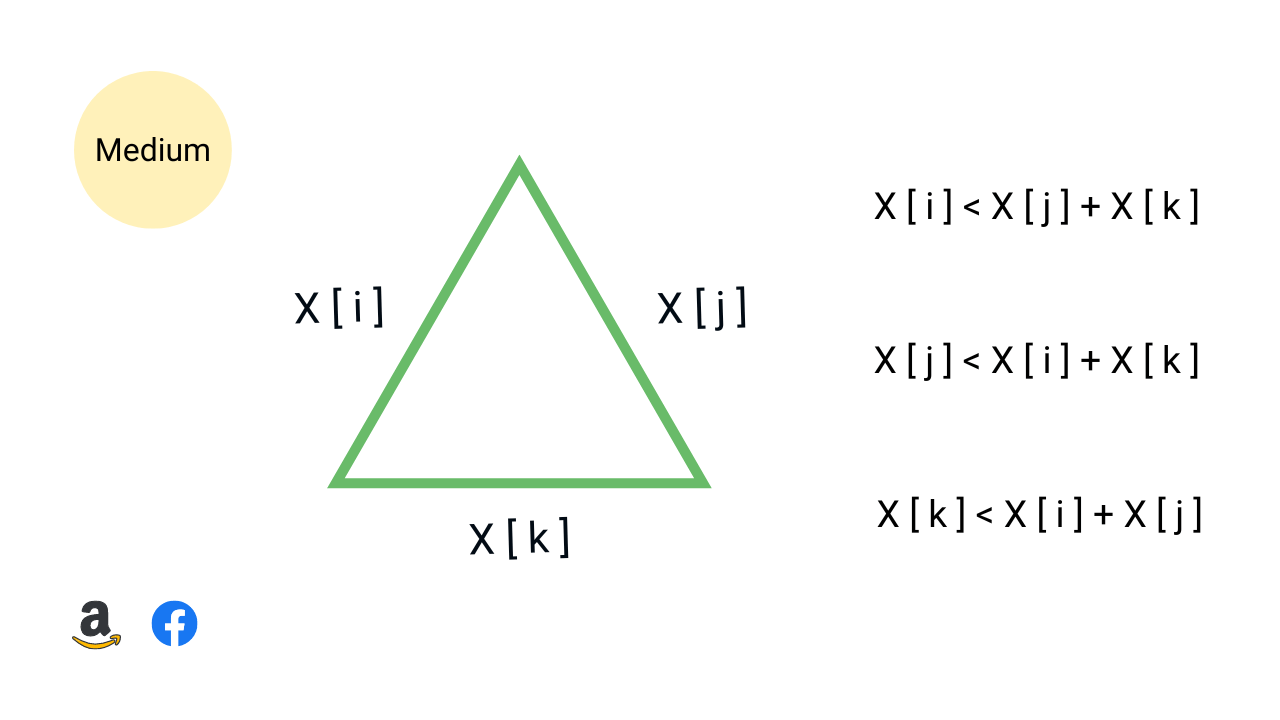Move Zeroes

Given an array X[] of n elements filled with several integers, some of them being zeroes, write a program to move all the zeros to the end. We need to preserve the relative order of non-zero elements and solve this problem in place using O(n) time complexity. Note: This is an excellent problem to learn problem solving using two pointers approach.Pair sum in an array

Given an array of n integers and a target number, write a program to find whether a pair sum exists in the array or not. In other words, we need to check for a pair of elements in the array that sum exactly to the target value. Assume that all elements are distinct. Note: This is an excellent problem to learn problem solving using two pointers and hash table.Find Intersection of Two Arrays

Given two integer arrays, X[] and Y[] of size m and n, write a program to find intersection of these two arrays. The intersection is a list of common elements present in both arrays. Suppose m > n, all array elements are distinct and intersection elements can be in any order. Note: This is an excellent problem to learn problem solving using various approaches.Most Frequent Element in an Array

Given an array X[] of size n, write a program to find the most frequent element in the array, i.e. the element which occurs the most number of times. If multiple elements have maximum frequency, return the smallest (assume that at least one element is repeated). Note: This is an excellent problem to learn problem-solving using sorting and hash table.Inorder Successor of a Node in Binary Search Tree (BST)

Given a node x and the root of a binary search tree, write a program to find the in-order successor of node x. The in-order successor of node x is a node that will be visited just after node x during an in-order traversal. In other words, the in-order successor of a node x is the node with the smallest key greater than x->key.Find Minimum and Maximum Element in an Array

Given an array X[] of size n, write a program to find the maximum and minimum elements while making the minimum number of comparisons. This is an excellent question to learn problem-solving using a single loop and divide and conquer approach. In the efficient single-loop solution, we increment the loop by two to optimize the comparison count.Count the number of possible triangles

Write a program to count the number of possible triangles that can be formed with three elements from a given unsorted array of positive integers. The three elements, representing the lengths of the sides of a triangle, must satisfy the triangle inequality: the sum of any two sides must be greater than the third side.Implement Queue Using Stacks

Write a program to implement queue using stack. We should use stack operations like push, pop, top, size, and isEmpty for implementing queue operations like enqueue, dequeue, and front. In this blog, we have discussed two approaches for implementing queue using two stacks: 1) Dequeue O(1) and Enqueue O(n) 2) Enqueue O(1) and Dequeue O(1).Remove Duplicates from Sorted Array

Given a sorted array, write a program to remove duplicates from the array. We need to remove repeated elements so that there is a single occurrence of each element and return the length of the array containing unique elements. Note: This is an excellent problem to learn the fast and slow pointers approach. We have discussed two O(n) time in-place solutions.Maximum Difference in an Array

Given an array A[] of n integers, find out the maximum difference between any two elements such that the larger element appears after the smaller element. In other words, we need to find max(A[j] - A[i]), where A[j] > A[i] and j > i. Note: This is an excellent problem to learn problem-solving using divide and conquer and a single loop.Find Triplets with Zero Sum

Given an array X[] of n distinct elements, write a program to find all the unique triplets in the array whose sum is equal to zero. For example, if triplets with zero sum in the array are (X[i], X[j], X[k]), then X[i] + X[j] + X[k] = 0. Hint: This is an excellent problem to learn problem-solving and optimization using hashing and two pointers approach.Container With Most Water

Given an array of n non-negative integers height[n], where each represents a point at coordinate (i, height[i]). n vertical lines are drawn such that endpoints of line i is at (i, height[i]) and (i, 0). Find two lines, which together with x-axis form a container, such that container contains the most water. This is an excellent problem to learn two pointers approach.Sort an Array of 0s, 1s and 2s (Dutch National Flag Problem)

Given an array consisting of 0s, 1s, and 2s, write a program to sort this array of 0, 1, and 2 in ascending order. We need to sort the array in O(n) time complexity without using sorting algorithms or extra space. Note: This is a variation of the Dutch national flag problem and an excellent problem to learn problem solving using three pointers.Product of array except self

Given an array X[] of n integers, write a program to find product[] array such that product[i] is equal to product of all array elements except X[i]. We need to solve this problem without using division operations. Note: This is an excellent product array puzzle to learn time complexity optimization using prefix array and a single loop.Rotate Matrix by 90 Degrees

Given an n x n 2D matrix, write a program to rotate the matrix by 90 degrees in the anticlockwise direction. The program should rotate the matrix 90 degrees without using extra space. In other words, we have to perform the rotation by modifying the 2D matrix directly. Note that this is an excellent problem to learn problem-solving using loops and the transpose of a matrix.Find Minimum in Rotated Sorted Array

A sorted and rotated array of size n is given, write a program to find minimum element in rotated sorted array. Rotation by k times means that the first k sorted elements of the array will move to the last k positions, and the last n - k sorted elements will move to the first n - k positions. Note: We don’t know how many times array is rotated in the given problem.Roman to Integer

Given a Roman number, write a program to convert Roman number to a corresponding integer value. What are Roman numbers? Roman numbers are the symbols used in a system of numerical notation based on the ancient Roman system. The symbols are I, V, X, L, C, D, and M, which represent integer values respectively for 1, 5, 10, 50, 100, 500, and 1,000.Minimum Depth of Binary Tree

Given a binary tree, find its minimum depth. The min depth of a binary tree is the number of nodes along the shortest path from root node down to the nearest leaf node. The path has to end on a leaf node. Note: An excellent problem to understand efficient problem solving using breadth-first search (BFS) when the solution node is nearest to the root node.